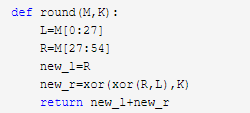# 护网杯一道密码学的感想

护网杯比赛，一道不算难的密码学却思路绕了好久才和出题人相符合，这里记录一下做题的过程及感想

题目的源码如下：

import os
def xor(a,b):
assert len(a)==len(b)
c=""
for i in range(len(a)):
c+=chr(ord(a[i])^ord(b[i]))
return c
def f(x,k):
return xor(xor(x,k),7)
def round(M,K):
L=M[0:27]
R=M[27:54]
new_l=R
new_r=xor(xor(R,L),K)
return new_l+new_r
def fez(m,K):
for i in K:
m=round(m,i)
return m

K=[]
for i in range(7):
K.append(os.urandom(27))
assert len(m)<54
m+=os.urandom(54-len(m))

test=os.urandom(54)
print test.encode("hex")
print fez(test,K).encode("hex")
print fez(m,K).encode("hex")

第二种思路：因为test是已知的，第一次fez的调用中用到了test和K，并且密文也是已知的，那么能不能解出来K，然后去解flag？于是回到round函数，可以看到最后一次加密结束时，用到的是K7,那么最后一次的E_L和E_R我们是知道的，我们能不能推出前一次的L和R，也就是没有经过轮加密的L和R，我们已经知道下一轮的密文L等于上一轮的R，下一轮的R等于上一轮的R异或L再异或当前轮所使用的K，那么很容易得出我们能获得上一轮所使用的明文的右半部分，有了上一轮的右半部分，加密后的新的右半部分，如果我们求出上一轮的左边的部分，就能求出当前轮所使用的K，但是根绝目前已知的所有条件，上一轮的明文的左半部分是无法求出的，卡在这里了很长时间，结果无奈放弃了第三种思路：正向来模拟加密的过程，来发现是否存在漏洞，在round函数中，新一轮的左半部分等于上一轮的右半部分，下一轮的右半部分等于上一轮的左右两部分异或之后再和当前轮的K异或，产生的新一轮的左右两部分拼接成为新的密文。

即假设初始明文为M，分为L和R两部分

1 第一轮：R+R^L^K1
2 第二轮：R^L^K1+R^R^L^K1=>R^L^K1+L^K1^K2
3 第三轮：L^K1^K2+R^K2^K3
4 第四轮：R^K2^K3+L^R^K1^K3^K4
5 第五轮：L^R^K1^K3^K4+L^K1^K2^K4^K5
6 第六轮：L^K1^K2^K4^K5+R^K2^K3^K5^k6
7 第七轮：R^K2^K3^K5^k6+R^L^K1^K3^K4^K6^K7

1.第七轮结束后的密文的左半部分异或后实际就是test变量的右半部分和flag的右半部分进行了一次异或，再和test本身的右半部分异或一次，就能得到m变量的右半部分。

2.第七轮结束后的密文的左右两部分异或得到只剩下原明文左半部分和K盒组成的结果，即两次fez加密的结果的各自的左右两部分先做异或运算，然后再把两组异或结果再一起异或一次，即为test变量的左半部分和m变量的左半部分异或的结果，又因为test变量已知，所以把结果再和test变量的左半部分异或一次就能得到m的左半部分。

1.先正向分析加密流程，分析加密后的结果组成，能不用暴力解决就不要用

2.逆向分析，从后往前推

posted @ 2018-10-14 18:11 tr1ple 阅读(...) 评论(...) 编辑 收藏